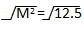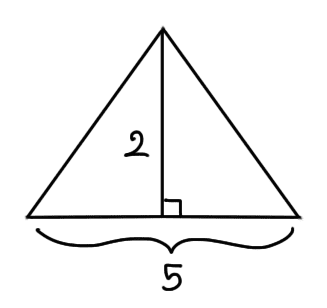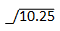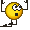# Finding areas of rectangles.

• Kirito123
In summary, the homework statement is trying to find the area of a rectangle, but is having trouble because it doesn't have the information for the height of the triangle. The attempt at a solution is to use the Pythagorean Theorem and to use the information that the OP knows, which is that the height is 2 and the base is 8. The final answer is that the length of the side of the rectangle is approximately 3.2m, and the area is 25.6.

## Homework Statement2. Homework Equations

A= l x w (area for a rectangle.

## The Attempt at a Solution

I will have to find the area of 1 halve of the roof, since there parallel I only have to find one side. So I know the height is 2 and I also know that the base of the rectangle is 8. I tried to find out but had no luck.

So my only problem is how do I find the other side of the rectangle?

#### Attachments

The height of the yellow rectangle is not, in fact, 2.

Its not?

Kirito123 said:
Its not?
Nope. That is the height of the triangle. There are completely different lengths. How do you think we can find the height of the rectangle?

I don't know but my guess is i have to find all the lengths of the triangle then i can answer the question.

The height of the rectangle is the side length of the triangle. You already know the height and base measurements for the triangle, which is all the information you need. Do you know how to find the side length of a triangle?

Draw end view to scale or use Pythagoras' Theorem

Nidum said:
Draw end view to scale
This is extremely inefficient, is it not?

The Pythagorean Theorem will work, but the OP was supposed to figure that out.

so i would use the formula a2 + b2= c2.

a2 + b2 = c2

m2 + m2 = 5m2 (Note: i am using m to represent the unknown lengths of the triangle)

2m2 = 25

2m2 / 2 = 25 / 2

m2 = 12.5m = 3.5

the length of the unknown sides is approximately 2.5 m

Correct?

Kirito123 said:
m2 + m2 = 5m2
This is wrong, though. In ##a^2+b^2=c^2##, do you know what the c stands for?

And for the Pythagorean Theorem to work, you need a right triangle.

c is the hypotenuse (the side opposite the 90° angle).

O do you mean that the triangle isn't a right angle?

Ok so that's why it won't work.

Kirito123 said:
O do you mean that the triangle isn't a right angle?
Kirito123 said:
Ok so that's why it won't work.
It CAN work. How would you "make" a right triangle for the Pythagorean Theorem to work? What values would you use?Break the triangle apart. You can still use the Pythagorean Theorem!

:O omg you just saved me 3 hours of searching... the fact that on the table above, i did that but didn't even notice... Ok ill answer it and repost to check if its right.

Please note that while the drawing seems to show that the roof is composed of two pieces of equal size, one of which is in yellow, there seems to be an unfounded assumption that the roof is composed of two identical pieces (one slanting down to the left, one slanting down to the right). All you know from the given information is that the length of the two triangles on one end (which share the red "height" line) sum to 5; the assumption that each is 2.5 is not justified. Another way of saying this is that the location of where the roof peak is (what distance from the front) is unspecified. I would solve this poorly specified problem by assuming the two pieces were of equal size and then for extra credit assume that one piece has 0 length (that is, the peak happens at the very back (making the one triangle on each end a right triangle.))

Ok so i got the answer for 1 halve of the triangle. I would divide 5 into 2 parts, so its become 2.5. I also have the height 2 for the triangle. Now i can use the Pythagorean Theorem to solve for the hypotenuse. a2 + b2 = c2.

2.5 x 2.5 = 6.25
2 x 2 = 4

6.25 + 4 = 10.25= 3.2

so the length of side of the rectangle would be approximately 3.2m.

•ProfuselyQuarky
That's it .

ok now i can find the area by my self THX ALOT guys.

Yay! You've done it! Knew you couldLet's see the finished answer, too.

ok i will thanks a lot your the man or the woman.

Kirito123 said:
ok i will thanks a lot your the man or the woman.
lol . . . not sure what you mean. I'm a 15 year old girlLol your very smart and then that means your the womanAnyway i got the area 25.6. A = l x w which is 8 x 3.2.

correct?

That's it! Just remember that the roof is made of two sides of that same rectangle so you have to multiple the product by 2.

I know, thanks a lot your really smart for a 15 year old if your REALLY are one.Kirito123 said:
I know, thanks a lot your really smart for a 15 year old if your REALLY are one.It has nothing to do with being smart or dumb. Just pay attention and use all the information that you've learned in the past. Now, no more posting--go back to doing your homework :P